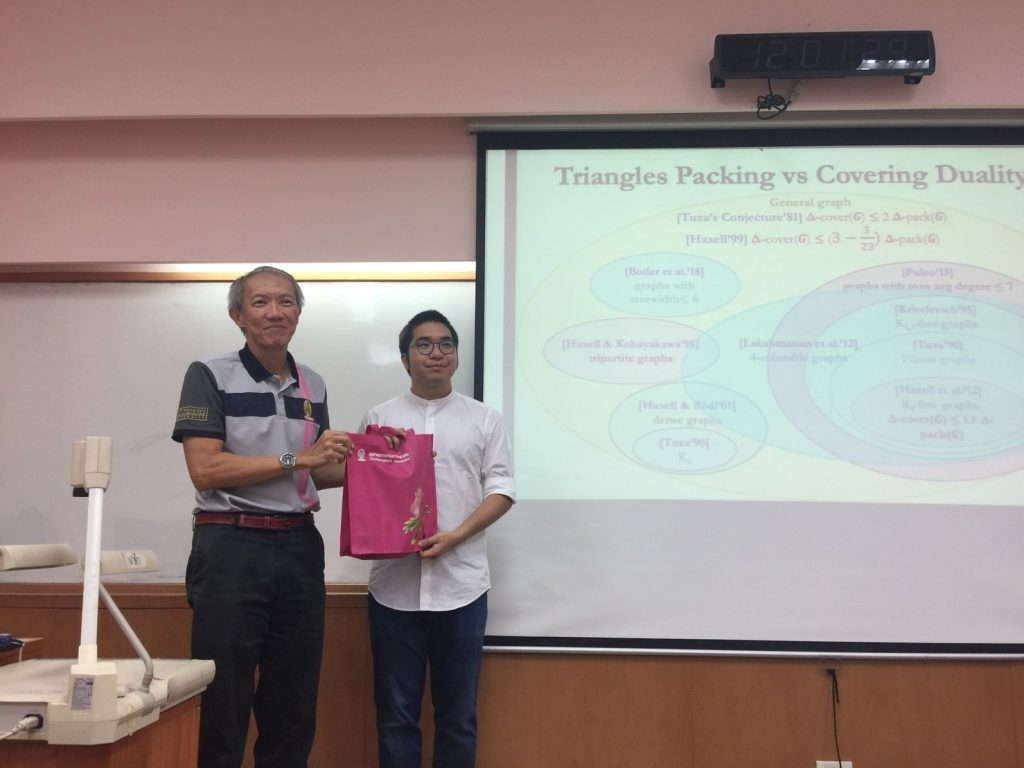# ภาพการบรรยายพิเศษ Title: Multi-transversals for Triangles and the Tuza’s Conjecture [SODA’ 20]

February 24, 2020   งานสัมมนา/ประชุมวิชาการ
ภาพการบรรยายพิเศษ Title: Multi-transversals for Triangles and
the Tuza’s Conjecture [SODA’ 20]

Pattara Sukprasert
Bio:
Pattara is a 3rd year Ph.D. student at Northwestern University where he is supervised by Samir Khuller. His research interest is on approximation algorithms on graph problems. He also has broad interests in algorithms and combinatorial optimization. Prior to this, he got his Bachelor’s degree from Chulalongkorn University and his Master’s degrees from Kasetsart University and The University of Maryland in College Park.
Title: Multi-transversals for Triangles and the Tuza’s Conjecture [SODA’ 20]

In this work, we study a primal and dual relationship about triangles: For any graph G, let ν(G) be the maximum number of edge-disjoint triangles in G, and τ(G) be the minimum subset F of edges such that G∖F is triangle-free. It is easy to see that ν(G)≤τ(G)≤3ν(G), and in fact, this rather obvious inequality holds for a much more general primal-dual relation between k-hyper matching and covering in hypergraphs. Tuza conjectured in 1981 that τ(G)≤2ν(G), and this question has received attention from various groups of researchers in discrete mathematics, settling various special cases such as planar graphs and generalized to bounded maximum average degree graphs, some cases of minor-free graphs, and very dense graphs. Despite these efforts, the conjecture in general graphs has remained wide open for almost four decades.

In this work, we provide a proof of a non-trivial consequence of the conjecture; that is, for every k≥2, there exist a (multi)-set F⊆E(G):|F|≤2kν(G) such that each triangle in G overlaps at least k elements in F. Our result can be seen as a strengthened statement of Krivelevich’s result on the fractional version of Tuza’s conjecture. The main technical ingredient of our result is a charging argument, that locally identifies edges in F based on a local view of the packing solution. This idea might be useful in further studying the primal-dual relations in general and the Tuza’s conjecture in particular.บรรยายพิเศษ วันที่ 24 กุมภาพันธ์ 2563